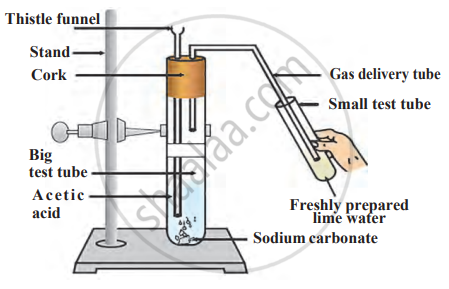# Write the answers to the questions by observing the following figure. 1. Write the chemical reaction shown in the figure above in the form of a balanced equation. - Science and Technology 2

Write the answers to the questions by observing the following figure.1. Write the chemical reaction shown in the figure above in the form of a balanced equation.
2. Write the name of the gas coming out of the large test tube in the above chemical reaction.
3. Why do small bubbles appear in the small test tube?
4. What is the change in colour of lime water?

#### Solution

1. Balanced equation of the reaction between acetic acid and sodium carbonate is as follows:
$\ce{\underset{\text{Acetic acid}}{2CH3COOH_{(aq)}} + \underset{\text{carbonate}}{\underset{\text{Sodium}}{Na2CO_{3(s)}}} -> \underset{\text{ethanoate}}{\underset{\text{Sodium}}{2CH3COONa_{(aq)}}} + \underset{\text{Water}}{H2O_{(l)}} + \underset{\text{Carbon dioxide}}{CO2\uparrow}}$
2. The gas formed in the large test tube is carbon dioxide (CO2) gas.
3. The carbon dioxide gas produced in big test tube passes through bent gas delivery tube to the small test tube containing lime water. Hence, bubbles are seen in the small test tube.
4. Lime water turns milky.
Concept: Ethanoic Acid
Is there an error in this question or solution?

Share